# The load, capacity, and availability of quorum systems

The load, capacity, and availability of quorum systems Naor & Wool, SIAM J Computing 1998

Update: fixed ‘non-intersection property’ to read ‘non-empty intersection property.’ Quite an important difference! With thanks to those who pointed out my mistake.

This is the paper that Howard et al referenced in Flexible Paxos as defining the “fundamental theorem of quorum intersection.” I like a good fundamental theorem, how could I possibly resist? When you first open up the pdf (or glance over your printout, if you’re that way inclined) it looks pretty daunting, but there’s a lot of great information in here once you dig in.

There are two main sections to the paper. First we are provided with some thinking tools and theorems for reasoning about quorum systems, and then the authors present four novel (as of 1998) quorum systems that have optimal or near optimal load, and high availability. As a bonus we get an analysis of some existing quorum systems too. If you’re looking for the proof that majority quorum systems are far from optimal, this is your paper…

### Reasoning about quorum systems

Let’s start out by defining a quorum system: a quorum system is a collection of sets S = {S1,S2,…Sm} with each S (called a quorum) drawn from some underlying universe U with cardinality n. Furthermore, for every S,R ∈ S, S and R have a non-empty intersection. This is the property that makes quorum systems useful: we are guaranteed to be able to pass an unbroken chain of information through such a system because at every step there is at least one participant that knows the ‘latest.’ The same property guarantees that a user will always have a consistent view of the system state.

There are lots of different schemes for guaranteeing the non-empty intersection property, the best-known and simplest of which is that every set S ∈ S must contain a majority of the members of U. We can evaluate these schemes using three primary criteria: the load it places on each member of the universe; the overall capacity of the system to handle requests; and the availability of the system, i.e. how resilient it is to failure.

#### Load

Take a quorum system that selects quorums from some universe of members U. There is a strategy in place for selecting quorums, which results in some load on each member of U – the number of times it is accessed.

The load(S) of a quorum system S is the minimal load on the busiest element.

In other words, no matter what strategy you come up with, there’s going to be at least one element that gets hit at least load(S) times.

If the minimum quorum size in a given system is denoted by cardinality(S), then:

load(S) ≥ max(1/cardinality(S) , cardinality(S)/n)

#### Capacity

We’d like the system to handle as many requests as possible. If a system is going to handle a accesses within a period of k time units, it’s clear that every element in U can be accessed at most k times.

By an analogy to hypergraph theory, the authors demonstrate that:

capacity(S) = 1/load(S)

And therefore all the information regarding the capacity of a quorum system is actually captured by knowing the load of the system (the minimal load on the busiest element of the system). This I believe is the fundamental theorem I alluded to at the start of this post.

#### Availability

If each element fails with probability p, we’re interested in the probability Fp that the surviving elements do not contain any quorum.

This failure probability measures how resilient the system is, and we would like Fp to be as small as possible.

If individual elements are more prone to failure, then the load of the system is higher. This trade-off is captured by the equation:

Fp ≥ pn.load(S)

The most interesting quorum systems will offer low load (and hence high capacity / throughput) coupled with high availability. Interestingly, when p < 1/2 then a majority is the best quorum system with respect to availability, yet it ‘fails miserably’ on load with a load score of 1/2 also. In fact, all voting systems have a load of at least 1/2, which is very high.

It’s tempting to use quorum size as a measure of load (i.e., intuitively it seems that systems requiring smaller quorum sizes should be better). But this turns out to be a mistake in isolation:

Several authors have emphasized the criterion of having small quorums. This is an important parameter since it captures the message complexity of a protocol using the quorum system. However, it does not tell us how to use the quorum so each element is used as infrequently as possible. Moreover, our lower bounds show that if the quorum size is small (ie. < √n) then decreasing it any further actually increases the load. We therefore argue that when analyzing a quorum system one should consider both its quorum size and load (and of course its availability) since each measures a different aspect of the system’s quality.

### The Paths quorum system

The paths quorum system is the best overall quorum system presented in the paper. It has a load of O(1/√n) and a failure probability e-Ω√n when elements fail with probability p < 1/2. Even in the presence of faults, the load is still O(1/&sqrt;n) with very high probability.

In the paths system each element (e.g. node) in the system is represented by an edge between two vertices is a specially constructed grid. A quorum system is then formed by joining the edges (elements) intersected by any left-to-right traversal of the grid with the edges (elements) intersected by any top-to-bottom traversal.

A Paths quorum system of order d has n = 2d2 + 2d + 1 elements. A quorum system involving 41 nodes therefore, would have d = 4 (and the minimum system size would be d=1 = 5 nodes).

Here’s how to make the grid (I’m going to do it for d = 1 since that’s quite complex enough, thank you!):

• Start off by laying out a d+1 x d+2 set of vertices, and create edges between them all except for the first and last columns, giving the grid G(d).
• Make a copy of that grid and rotate it counter-clockwise by 90 degrees, giving G*(d)
• Superimpose G*(d) on G(d) at offset 1/2,-1/2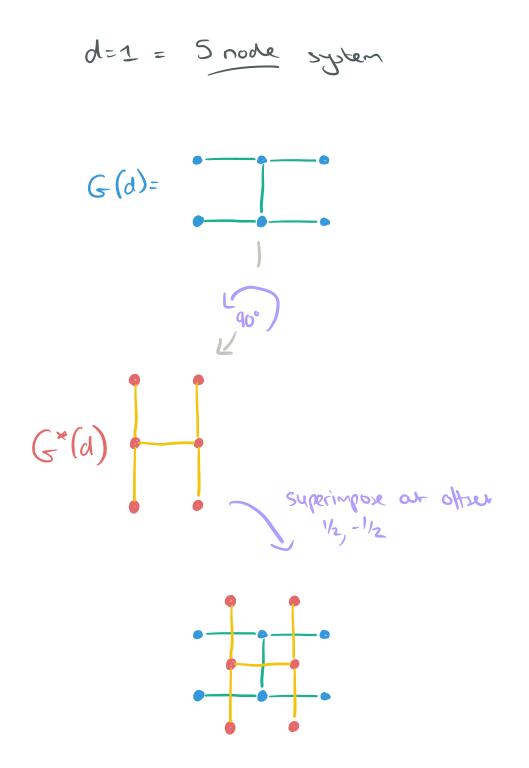• Still with me? Here come’s the part that feels really counter-intuitive to me. You see, I really want those vertices to represent my nodes (that’s what happens in just about every other diagram of this kind right?), but they don’t. Each node is represented by the point where an edge from G(d) crosses an edge from G*(d):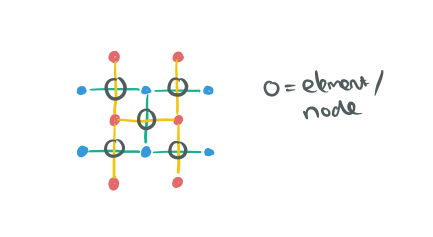• To form a quorum, take any path along edges crossing the grid from left-right, and any path along edges crossing the grid from top-to-bottom. Your quorum members are the elements (nodes) that sit in the edges you traversed: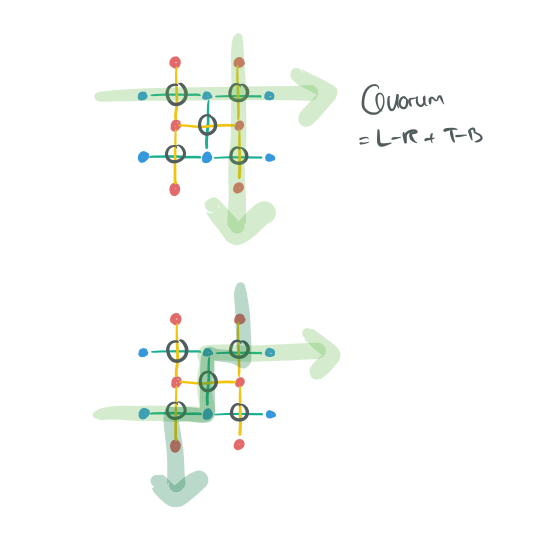I admire the theoretical properties of this construction, but I also find this really hard to think about when it comes to creating a practical implementation. Is it just me? I went well over my paper time budget trying to get my understanding to a point where I could explain it relatively simply. Even then, I’m not sure if I succeeded but I’ll let you be the judge of that!

If you did want to implement this, the authors kindly describe an efficient algorithm that finds a nearly optimal quorum selection strategy w for any given configuration x. The load induced by w is no more than twice the optimal load for x. Here goes:

As a preprocessing step, that needs to be performed after each configuration change, the algorithm finds a maximum collection of disjoint left-right paths, say kLR such paths, and similarly finds kTM disjoint top-bottom paths. This can be done by connecting a source vertex s to all the vertices on the left side and a sink t to all the vertices on the right, assigning a capacity of 1 to all the edges, and finding the maximum (s,t) flow (and repeating for TB paths). Since the network is planar we can find the flow in time O(n log n) using the algorithm of , or in time O(n √ log n) by  using the methods of . Given these path collections, the strategy w is the following: If either kLR or _kTB = 0 then no live configurations exist in configuration x. Otherwise, whenever a quorum is needed, pick a LR path with uniform probability 1/kLR, and a TB path with uniform probability 1/kTB and use their union.

That feels like quite a lot of complexity to drop into e.g. the middle of an already pretty complex consensus algorithm though – especially compared to a good old majority quorum system!

The other three strategies are easier to understand, and I shall give just a very brief treatment of one of them, the B-Grid quorum system.

### The B-Grid quorum system

Arrange elements in a rectangular grid of width d, and split the grid into h bands of r rows each. For n nodes we have n = dhr. Each element is represented by a square in the grid.

To form a quorum take one mini-column in every band, and then add a representative element from every mini-column of one band: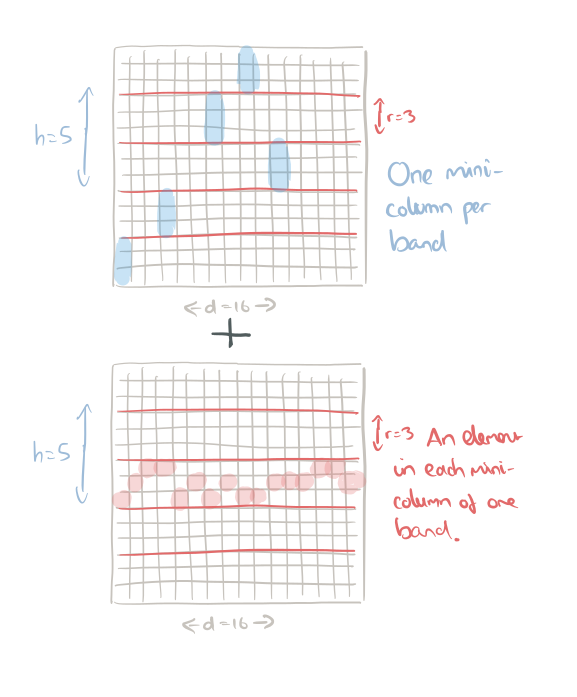You’ll end up with something like this: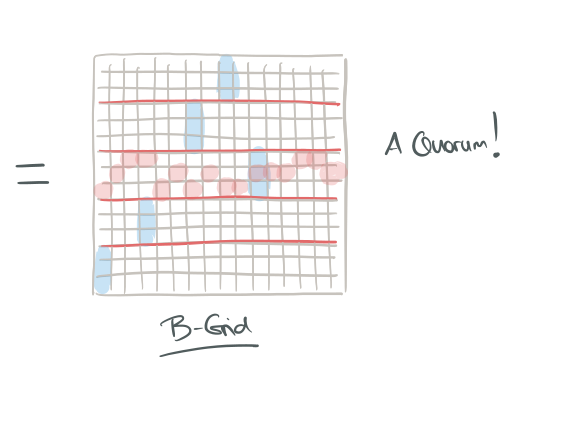The example above is a B-Grid with 240 elements, d = 16, h = 5, r = 3.

The load is given by (d + hr – 1) / dhr. The precise formula for failure probability depends on the value of d and its relation to n. Choosing d > n2/3 or d < √n makes both load and availability worse. Within this range of d, Fp is O(e-n2/3) when the load is ~ n-1/3.